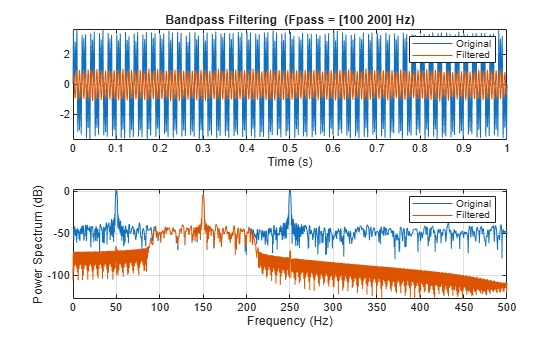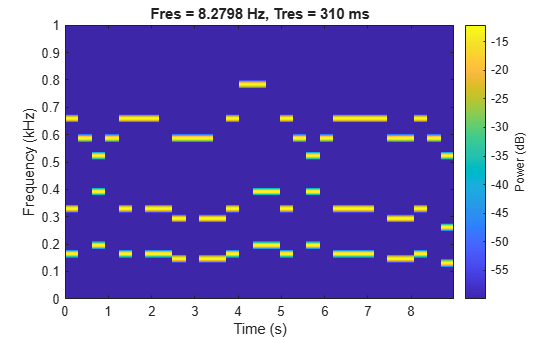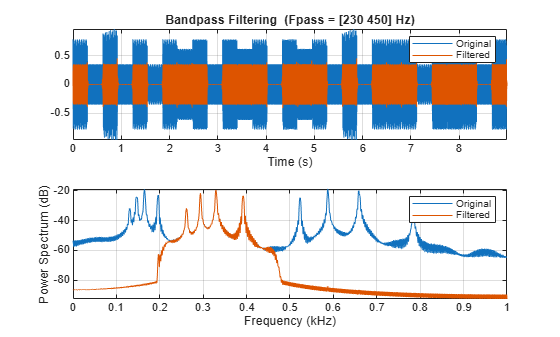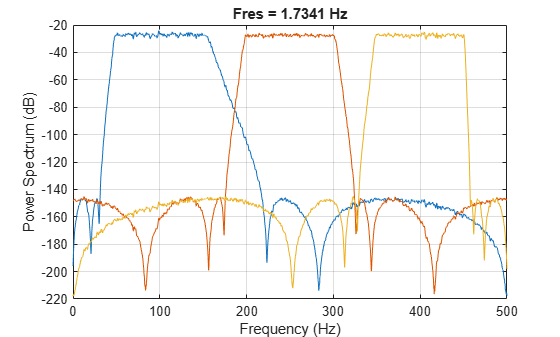# bandpass

Bandpass-filter signals

## Syntax

``y = bandpass(x,wpass)``
``y = bandpass(x,fpass,fs)``
``y = bandpass(xt,fpass)``
``y = bandpass(___,Name,Value)``
``[y,d] = bandpass(___)``
``bandpass(___)``

## Description

````y = bandpass(x,wpass)` filters the input signal `x` using a bandpass filter with a passband frequency range specified by the two-element vector `wpass` and expressed in normalized units of π rad/sample. `bandpass` uses a minimum-order filter with a stopband attenuation of 60 dB and compensates for the delay introduced by the filter. If `x` is a matrix, the function filters each column independently.```

example

````y = bandpass(x,fpass,fs)` specifies that `x` has been sampled at a rate of `fs` hertz. The two-element vector `fpass` specifies the passband frequency range of the filter in hertz.```
````y = bandpass(xt,fpass)` bandpass-filters the data in timetable `xt` using a filter with a passband frequency range specified in hertz by the two-element vector `fpass`. The function independently filters all variables in the timetable and all columns inside each variable.```

example

````y = bandpass(___,Name,Value)` specifies additional options for any of the previous syntaxes using name-value pair arguments. You can change the stopband attenuation, the Bandpass Filter Steepness, and the type of impulse response of the filter.```

example

````[y,d] = bandpass(___)` also returns the `digitalFilter` object `d` used to filter the input.```
````bandpass(___)` with no output arguments plots the input signal and overlays the filtered signal.```

## Examples

collapse all

Create a signal sampled at 1 kHz for 1 second. The signal contains three tones, one at 50 Hz, another at 150 Hz, and a third at 250 Hz. The high-frequency and low-frequency tones both have twice the amplitude of the intermediate tone. The signal is embedded in Gaussian white noise of variance 1/100.

```fs = 1e3; t = 0:1/fs:1; x = [2 1 2]*sin(2*pi*[50 150 250]'.*t) + randn(size(t))/10;```

Bandpass-filter the signal to remove the low-frequency and high-frequency tones. Specify passband frequencies of 100 Hz and 200 Hz. Display the original and filtered signals, and also their spectra.

`bandpass(x,[100 200],fs)`Implement a basic digital music synthesizer and use it to play a traditional song. Specify a sample rate of 2 kHz. Plot the spectrogram of the song.

```fs = 2e3; t = 0:1/fs:0.3-1/fs; l = [0 130.81 146.83 164.81 174.61 196.00 220 246.94]; m = [0 261.63 293.66 329.63 349.23 392.00 440 493.88]; h = [0 523.25 587.33 659.25 698.46 783.99 880 987.77]; note = @(f,g) [1 1 1]*sin(2*pi*[l(g) m(g) h(f)]'.*t); mel = [3 2 1 2 3 3 3 0 2 2 2 0 3 5 5 0 3 2 1 2 3 3 3 3 2 2 3 2 1]+1; acc = [3 0 5 0 3 0 3 3 2 0 2 2 3 0 5 5 3 0 5 0 3 3 3 0 2 2 3 0 1]+1; song = []; for kj = 1:length(mel) song = [song note(mel(kj),acc(kj)) zeros(1,0.01*fs)]; end song = song/(max(abs(song))+0.1); % To hear, type sound(song,fs) pspectrum(song,fs,'spectrogram','TimeResolution',0.31, ... 'OverlapPercent',0,'MinThreshold',-60)```Bandpass-filter the signal to separate the middle register from the other two. Specify passband frequencies of 230 Hz and 450 Hz. Plot the original and filtered signals in the time and frequency domains.

```pong = bandpass(song,[230 450],fs); % To hear, type sound(pong,fs) bandpass(song,[230 450],fs)```Plot the spectrogram of the middle register.

```figure pspectrum(pong,fs,'spectrogram','TimeResolution',0.31, ... 'OverlapPercent',0,'MinThreshold',-60)```Filter white noise sampled at 1 kHz using an infinite impulse response bandpass filter with a passband width of 100 Hz. Use different steepness values. Plot the spectra of the filtered signals.

```fs = 1000; x = randn(20000,1); [y1,d1] = bandpass(x,[ 50 150],fs,'ImpulseResponse','iir','Steepness',0.5); [y2,d2] = bandpass(x,[200 300],fs,'ImpulseResponse','iir','Steepness',0.8); [y3,d3] = bandpass(x,[350 450],fs,'ImpulseResponse','iir','Steepness',0.95); pspectrum([y1 y2 y3],fs) legend('Steepness = 0.5','Steepness = 0.8','Steepness = 0.95', ... 'Location','south')```Compute and plot the frequency responses of the filters.

```[h1,f] = freqz(d1,1024,fs); [h2,~] = freqz(d2,1024,fs); [h3,~] = freqz(d3,1024,fs); plot(f,mag2db(abs([h1 h2 h3]))) legend('Steepness = 0.5','Steepness = 0.8','Steepness = 0.95', ... 'Location','south') ylim([-100 10])```Make the filters asymmetric by specifying different values of steepness at the lower and higher passband frequencies.

```[y1,d1] = bandpass(x,[ 50 150],fs,'ImpulseResponse','iir','Steepness',[0.5 0.8]); [y2,d2] = bandpass(x,[200 300],fs,'ImpulseResponse','iir','Steepness',[0.5 0.8]); [y3,d3] = bandpass(x,[350 450],fs,'ImpulseResponse','iir','Steepness',[0.5 0.8]); pspectrum([y1 y2 y3],fs)```Compute and plot the frequency responses of the filters.

```[h1,f] = freqz(d1,1024,fs); [h2,~] = freqz(d2,1024,fs); [h3,~] = freqz(d3,1024,fs); plot(f,mag2db(abs([h1 h2 h3]))) ylim([-100 10])```## Input Arguments

collapse all

Input signal, specified as a vector or matrix.

Example: `sin(2*pi*(0:127)/16)+randn(1,128)/100` specifies a noisy sinusoid

Example: `[2 1].*sin(2*pi*(0:127)'./[16 64])` specifies a two-channel sinusoid.

Data Types: `single` | `double`
Complex Number Support: Yes

Normalized passband frequency range, specified as a two-element vector with elements in the interval (0, 1).

Passband frequency range, specified as a two-element vector with elements in the interval (0, `fs`/2).

Sample rate, specified as a positive real scalar.

Input timetable. `xt` must contain increasing, finite, and equally spaced row times of type `duration` in seconds.

If a timetable has missing or duplicate time points, you can fix it using the tips in Clean Timetable with Missing, Duplicate, or Nonuniform Times.

Example: `timetable(seconds(0:4)',randn(5,1),randn(5,2))` contains a single-channel random signal and a two-channel random signal, sampled at 1 Hz for 4 seconds.

### Name-Value Arguments

Specify optional comma-separated pairs of `Name,Value` arguments. `Name` is the argument name and `Value` is the corresponding value. `Name` must appear inside quotes. You can specify several name and value pair arguments in any order as `Name1,Value1,...,NameN,ValueN`.

Example: `'ImpulseResponse','iir','StopbandAttenuation',30` filters the input using a minimum-order IIR filter that attenuates by 30 dB the frequencies smaller than `fpass(1)` and the frequencies larger than `fpass(2)`.

Type of impulse response of the filter, specified as the comma-separated pair consisting of `'ImpulseResponse'` and `'fir'`, `'iir'`, or `'auto'`.

• `'fir'` — The function designs a minimum-order, linear-phase, finite impulse response (FIR) filter. To compensate for the delay, the function appends to the input signal N/2 zeros, where N is the filter order. The function then filters the signal and removes the first N/2 samples of the output.

In this case, the input signal must be at least twice as long as the filter that meets the specifications.

• `'iir'` — The function designs a minimum-order infinite impulse response (IIR) filter and uses the `filtfilt` function to perform zero-phase filtering and compensate for the filter delay.

If the signal is not at least three times as long as the filter that meets the specifications, the function designs a filter with smaller order and thus smaller steepness.

• `'auto'` — The function designs a minimum-order FIR filter if the input signal is long enough, and a minimum-order IIR filter otherwise. Specifically, the function follows these steps:

• Compute the minimum order that an FIR filter must have to meet the specifications. If the signal is at least twice as long as the required filter order, design and use that filter.

• If the signal is not long enough, compute the minimum order that an IIR filter must have to meet the specifications. If the signal is at least three times as long as the required filter order, design and use that filter.

• If the signal is not long enough, truncate the order to one-third the signal length and design an IIR filter of that order. The reduction in order comes at the expense of transition band steepness.

• Filter the signal and compensate for the delay.

Transition band steepness, specified as the comma-separated pair consisting of `'Steepness'` and a scalar or two-element vector with elements in the interval [0.5, 1). As the steepness increases, the filter response approaches the ideal bandpass response, but the resulting filter length and the computational cost of the filtering operation also increase. See Bandpass Filter Steepness for more information.

Filter stopband attenuation, specified as the comma-separated pair consisting of `'StopbandAttenuation'` and a positive scalar in dB.

## Output Arguments

collapse all

Filtered signal, returned as a vector, a matrix, or a timetable with the same dimensions as the input.

Bandpass filter used in the filtering operation, returned as a `digitalFilter` object.

collapse all

### Bandpass Filter Steepness

The `'Steepness'` argument controls the width of a filter's transition regions. The lower the steepness, the wider the transition region. The higher the steepness, the narrower the transition region.

To interpret the filter steepness, consider the following definitions:

• The Nyquist frequency, fNyquist, is the highest frequency component of a signal that can be sampled at a given rate without aliasing. fNyquist is 1 (×π rad/sample) when the input signal has no time information, and `fs`/2 hertz when the input signal is a timetable or when you specify a sample rate.

• The lower and upper stopband frequencies of the filter, fstoplower and fstopupper, are the frequencies below which and above which the attenuation is equal to or greater than the value specified using `'StopbandAttenuation'`.

• The lower transition width of the filter, Wlower, is `fpass`lowerfstoplower, where the lower passband frequency `fpass`lower is the first element of the specified `fpass`.

• The upper transition width of the filter, Wupper, is fstopupper`fpass`upper, where the upper passband frequency`fpass`upper is the second element of `fpass`.

• Most nonideal filters also attenuate the input signal across the passband. The maximum value of this frequency-dependent attenuation is called the passband ripple. Every filter used by `bandpass` has a passband ripple of 0.1 dB.To control the width of the transition bands, you can specify `'Steepness'` as either a two-element vector, [slower,supper], or a scalar. When you specify `'Steepness'` as a vector, the function:

• Computes the lower transition width as

Wlower = (1 – slower) × `fpass`lower.

• When the first element of `'Steepness'` is equal to 0.5, the transition width is 50% of `fpass`lower.

• As the first element of `'Steepness'` approaches 1, the transition width becomes progressively narrower until it reaches a minimum value of 1% of `fpass`lower.

• Computes the upper transition width as

Wupper = (1 – supper) × (fNyquist`fpass`upper).

• When the second element of `'Steepness'` is equal to 0.5, the transition width is 50% of (fNyquist`fpass`upper).

• As the second element of `'Steepness'` approaches 1, the transition width becomes progressively narrower until it reaches a minimum value of 1% of (fNyquist`fpass`upper).

When you specify `'Steepness'` as a scalar, the function designs a filter with equal lower and upper transition widths. The default value of `'Steepness'` is 0.85.

### Functions

Introduced in R2018a